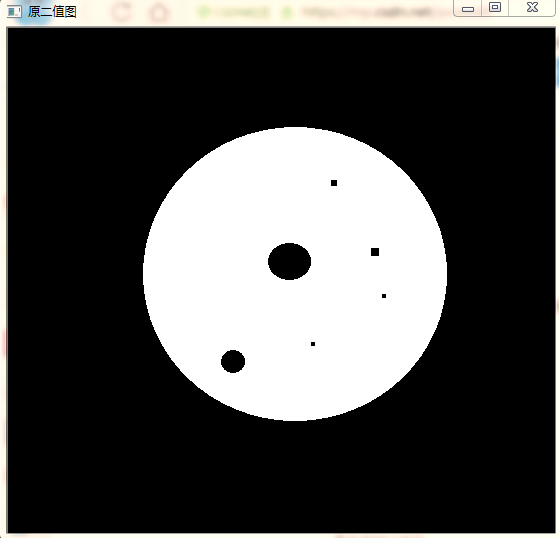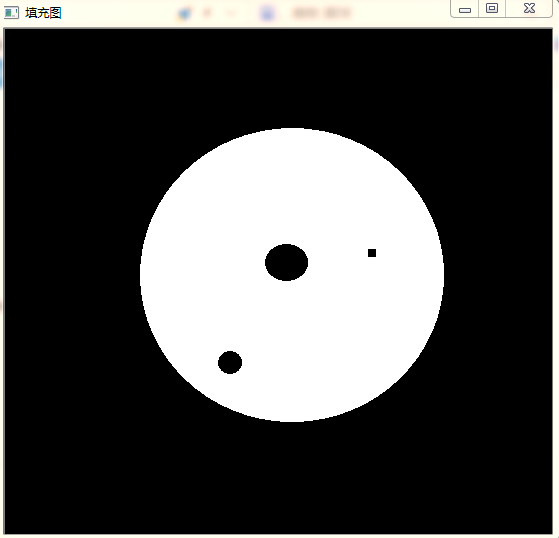# 图像处理孔洞填充

## 二值图像孔洞填充

2017-10-04 17:14:21 qq_30311601 阅读数 9488
• ###### 书本封面文字识别

学完这门课程可以掌握C#数据库信息管理技巧和方法 掌握chart图表控件的使用 Excel数据的导出 两个日期间的数据查询，并生成图表

3人学习 金圣韬
免费试看

（这里的二值为0和255）

1.确定二值图像像素[0,0]为初始种子点，这里认为[0,0]像素点为背景点，而非某个孔洞内部的点。

2.以种子点为起点，采用形态学膨胀算法对背景进行填充。膨胀到不能膨胀为止。膨胀运算采用四连通结构元。

3.背景填充结束后，对得到的二值图像取反得到新的二值图像，此时图像为全部孔洞的点集。

4.将第三步骤得到的二值图像与原二值图像相加及得到孔洞填充的结果。## opencv-python图像孔洞填充

2018-11-24 16:26:09 dugudaibo 阅读数 6625
• ###### 书本封面文字识别

学完这门课程可以掌握C#数据库信息管理技巧和方法 掌握chart图表控件的使用 Excel数据的导出 两个日期间的数据查询，并生成图表

3人学习 金圣韬
免费试看

在进行图像分割的过程中，由于算法的不稳定或者图像质量的问题，会造成图像孔洞出现，这个时候就需要对图像中的孔洞进行填充，具体函数如下

def fillHole(im_in):
im_floodfill = im_in.copy()

# Mask used to flood filling.
# Notice the size needs to be 2 pixels than the image.
h, w = im_in.shape[:2]

# Floodfill from point (0, 0)

# Invert floodfilled image
im_floodfill_inv = cv2.bitwise_not(im_floodfill)

# Combine the two images to get the foreground.
im_out = im_in | im_floodfill_inv

return im_out


这里调用了 opencv 库中的泛洪函数。我们将图像输入就可以获得整幅图像填充后的结果。

## 二值图像中封闭孔洞的高效填充算法（附源码）。

2013-09-08 11:30:00 weixin_33924220 阅读数 897
• ###### 书本封面文字识别

学完这门课程可以掌握C#数据库信息管理技巧和方法 掌握chart图表控件的使用 Excel数据的导出 两个日期间的数据查询，并生成图表

3人学习 金圣韬
免费试看

写具体类容之前先吐槽一下。

我一直写技术文档，虽然水平不怎么样，但是基本上我写的都还是比较实际的东西，也是自己投入了很多精力做的东西。有些可能没有开源，有些人觉得对他没有什么帮助，而我认为真正做技术的有能力的对于业内的东西，面对没有处理过的问题，只要有人随便点拨一下，基本上就能够找到解决方案，授人以渔而非授人以鱼才是重点。不过我心里不平衡的一点就是，无论是在CSDN还是博客园，得到人们参与评价和推荐的最多的很少有纯技术性的文章出现，像我这样从不写些八卦的东西的，觉得很是失望。

不排除我写的东西比较浅显，或者用词不合适，不能满足读者的口味，不过还是希望博客园及广大的博主能对技术性的博文多一份关心、多一份支持。

鉴于心情不好，这篇文章只是简单的说说这个算法的过程。

在对图像二值化后，不管用的是什么二值算法，总会存在一些瑕疵，这个时候我们就需要进行一些列的处理，去除那些我们不想要的糟粕，这类方法其实有很多，比如去除孤点、去除孤枝等等，这里介绍下去除封闭孔洞的一种算法。

首先，注意我们这里是去除封闭孔洞，何谓封闭孔洞？我们认为如果一个特征的边缘完全被另外一个特征包围，则认为其为一个封闭的特征，比如在下图中：1所标注处就是封闭的孔洞，2所标注极为开式孔洞。

对于识别来说，很多情况下，我们希望能够把这些封闭孔洞用周边的特征来填充，从而减少特征的数量。

一种直觉的想法就是，用FloodFill，不过如果直接用FloodFill，我们无法直接定位那些未知需要进行种子填充的， 但是Gabriel Landini, G.Landini 在2008年5月给我们写了个非常简单的代码实现了这一过程(原始代码是JAVA的，话说JAVA的算法代码改为C#基本就不要做什么改动啊）：

    public static void FillHole(FastBitmap Bmp)
{
int X, Y;
int Width,Height,Stride;
byte * Pointer;
Width = Bmp.Width; Height = Bmp.Height; Pointer = Bmp.Pointer; Stride = Bmp.Stride;
for (Y=0;Y<Height;Y++)
{
Pointer=Bmp.Pointer + Y*Stride;
if (Pointer==0) FloodFill(Bmp,0,Y);
if (Pointer[Width-1]==0) FloodFill(Bmp,Width-1,Y);
}
for (X=0;X<Width;X++)
{
Pointer=Bmp.Pointer + X;
if (Pointer==0) FloodFill(Bmp,X,0);
if (Pointer[(Height-1)*Stride]==0) FloodFill(Bmp,X,Height-1);
}
for (Y = 0; Y < Height; Y++)
{
Pointer = Bmp.Pointer + Y * Stride;
for (X = 0; X < Width; X++)
{
if (Pointer[X] == 127)
Pointer[X] = 0;
else
Pointer[X] = 255;
}
}
}

算法的过程很简单，先水平方向取起点和终点为种子点，进行种子填充，然后再垂直方向进行。不要以为需要有那么多次种子填充的过程，算法速度就很慢，由于在每次种子填充前，都有个判断条件，而该判断条件，随着前面种子填充的过程的进行，将越来越难以满足。
算法具体的原理留给有兴趣的人思考，直接使用的人就完全不用去管他，知道他有这个功能就OK了。

关于FloodFill算法的实现，多少年来也不知道有多少个版本的代码，能从网上找到的99%的都是些垃圾代码，真正的优秀代码作者一般都会留着，我这也是从网上找了一段代码，敷衍了事把，虽然我这里有非常好的这个函数。愿意学习的自然会去改进的。

下面我们来看一下填充的效果：原图　　　　　　　　　　　　　　　　　　　　　　　 二值图　　　　　　　　　　　　　　　　　　        　填充后的图

至于是要填充掉前景的孔洞还是背景的孔洞这可能需要作者自己判断了。

如果我们要去掉指定面积小于指定值得孔洞，而保留大于的，你知道该怎么办吗？

关于FloodFill函数，我在稍微展开一下吧，一般情况下这个函数都是用的四领域或者八领域的区域生长法实现的，如果能充分掌握该函数的编写，可以实现很多功能，比如PS的连续的魔术棒功能、比如二值图像的去除噪点、漫画分割、一些识别上等等，举例如下：

一、连续的魔术棒二、清除二值图像的孤点是不是感觉和这里的填充孔洞类似，不过两者还是有所区别的。

三、PCB板的某个元器件的定位好了，不扩展了，对填充孔洞有兴趣的朋友可以从这里下载源码：http://files.cnblogs.com/Imageshop/FillHole.rar

希望看过认为好的朋友多多支持。

*********************************作者： laviewpbt   时间： 2013.9.8       联系QQ:  33184777  转载请保留本行信息************************

## 数字图像处理（作业三）——孔洞填充+全局阈值+自适应阈值

2019-12-13 21:58:44 weixin_44586750 阅读数 270
• ###### 书本封面文字识别

学完这门课程可以掌握C#数据库信息管理技巧和方法 掌握chart图表控件的使用 Excel数据的导出 两个日期间的数据查询，并生成图表

3人学习 金圣韬
免费试看

## 一、孔洞填充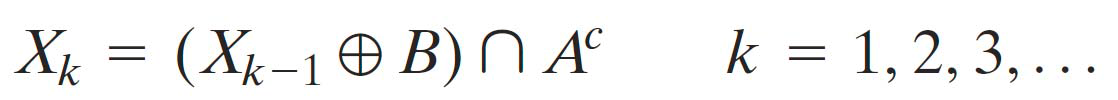1.首先找出所有孔洞的位置，只需知道洞中的一个点的坐标即可，下面直接以改点代替该洞
2.新建一张全零图，用0表示背景，1表示前景，大小与原图相同
（1）取出一个洞的坐标，在新图中该位置表1
（2）对该新图用一个结构元进行膨胀，然后再与原图的反（孔洞的地方应全为1）求与
（3）如果检测到一次操作完的结果与操作前相同，则结束迭代
（4）取出下一个洞的坐标，返回到（2）操作，直到所有洞都补完
3.此时新图中为1的位置即为需要填补的孔洞，将新图与原图相或即得到孔洞填充完成的图像

clc;clear;
BW=im2bw(A);
AC=~BW;
[m,n]=size(BW);
T=zeros(m,n);

%膨胀操作 某位置只要与结构元有交际，则该位置就为集合
%x0找出了所有的孔洞位置
x0={[29,24],[92,20],[187,17],[48,79],[2,91],[132,72],[92,102],[236,70],[213,117],[53,153],[122,155],[197,189],[262,181],[29,230],[119,233],[204,240],[260,250]};
for i=1:length(x0)
flag=1;
T_last=zeros(m,n);
col_temp=x0{i}(1);
row_temp=x0{i}(2);

T(row_temp,col_temp)=1; 	  %令该位置为1 然后利用模板进行膨胀
while flag

T=imdilate(T,SE);
T=T&AC;               %将T与背景相与，注意这里T和AC用1表示需要填充的空洞即黑色局域

if T==T_last          %如果操作前后没有变化则认为填充完成
flag=0;
end
T_last=T;
end
end
New=BW|T;

edge=New-imerode(New,SE);

subplot(131);
imshow(BW);title('处理前');
subplot(132);
imshow(New);title('处理后');
subplot(133);
imshow(edge);title('边缘');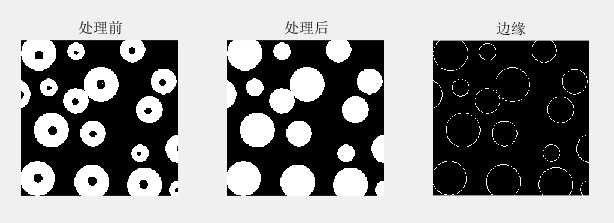## 2.1 迭代阈值

Ti1=(小于Ti的平均灰度+大于Ti的平均灰度)/2，一直迭代到Ti1与Ti相同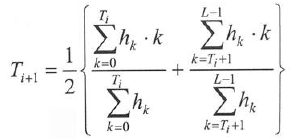matlab默认操作的数据类型是double，所以转int时一定要慎重，一个表达式中有一个int整个表达式就是int型了！

clc;clear;
A=rgb2gray(A);

H=imhist(A);
h=H./sum(H);
h=h';
T0=120;
error=100;

%循环或用矩阵相乘做
%这里注意表达式中存在int整个式子就默认为int型
while (error)>=1e-5
temp1=0;
sum1=sum(h(1:(T0+1)));
temp2=0;
sum2=sum(h((T0+2):256));
for i=1:double(T0+1)
temp1=temp1+h(i)*(i-1)/sum1;
end

%     x=double(0:T0);
%     temp1=x*h(1:T0+1)'/sum(h(1:T0+1));

for j=double(T0+2):256
temp2=temp2+h(j)*(j-1)/sum2;
end
%     y=double(T0+1:255);
%     temp2=y*h(T0+2:256)'/sum(h(T0+2:256));

T1=(1/2*(temp1+temp2));
error=abs(T1-T0);
T0=int32(T1);
end

% while error>1
%     temp1=(find(A>T0));
%     temp2=(find(A<T0));
%     T1=1/2*(mean(A(temp1))+mean(A(temp2)));
%     error=abs(T1-T0);
%     T0=T1;
% end

[m,n]=size(A);
A1=A;
for i=1:m
for j=1:n
if A1(i,j)>T0
A1(i,j)=255;
else
A1(i,j)=0;
end
end
end
str1=['迭代法 阈值为',num2str(T0)];
subplot(121);
imshow(A1);title(str1);


## 2.2 Otsu方法, 大津法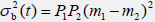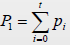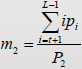%otsu方法
for i=1:256
P1=sum(h(1:i));
P2=1-P1;
m1=0;
m2=0;
for j=1:256
if j<=i
m1=m1+h(j)*(j-1)/P1;
else
m2=m2+h(j)*(j-1)/P2;
end
end

op(i)=P1*P2*(m1-m2)^2;
end
t=find(op==max(op))-1;
A2=A;
for i=1:m
for j=1:n
if A2(i,j)>t
A2(i,j)=255;
else
A2(i,j)=0;
end
end
end
str2=['大津法 阈值为',num2str(t)];
subplot(122);
imshow(A2);title(str2);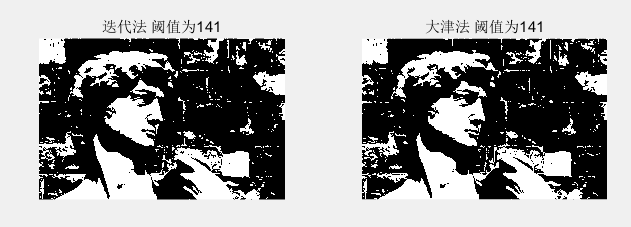## 三、自适应阈值

1.将整幅图像分成一系列互相有50%重叠的子图像；
2.得到每个子图像的直方图；
3.检测各子图像是否为双峰，若是则采用最优阈值法，否则不进行处理；
4.根据对直方图为双峰的子图像得到的阈值，通过插值得到所有子图像的插值；
5.根据各子图像的阈值，然后对图像进行分割；

1.这里判断双峰采用了findpeaks()函数，并规定而间距过小的峰值不算，检测到的极大值的个数超过1个则认为是双峰；
2.插值利用的是imresize()函数，直接将得到的一系列阈值模板插值得到与原图等大的阈值矩阵；
3.这里处理的具体方法为：
（1）先不管峰的个数，直接利用大津法直接得到阈值模板后插值得到阈值矩阵T；
（2）对峰的个数进行判断，如果仅有1个的话该处阈值设为0，得到阈值模板后进行插值得到阈值矩阵T1；
（3）最终的阈值模板应为T2=(T1&T).*T

clc;clear;
A=rgb2gray(A);
[m1,n1]=size(A);
row_num=floor(m1/5);
col_num=floor(n1/5);
for i=0:(row_num-2)
for j=0:(col_num-2)
block=A(1+5*i:1+5*i+9,1+5*j:1+5*j+9);
B=imhist(block);
pks=findpeaks(B,'minpeakdistance',35);
if length(pks)>1
t1=255*graythresh(block);
else
t1=0;
end
t=255*graythresh(block);
T(i+1,j+1)=t;
T1(i+1,j+1)=t1;
end
end
T_1=imresize(T,[m1,n1],'bilinear');
T1_1=imresize(T1,[m1,n1],'bilinear');
T2=T_1&T1_1;
T2_1=T2.*T_1;
for i=1:m1
for j=1:n1
if A(i,j)>T2_1(i,j)
A(i,j)=255;
else
A(i,j)=0;
end
end
end

figure;
%进行开操作
A=imerode(A,SE);
A=imdilate(A,SE)
imshow(A);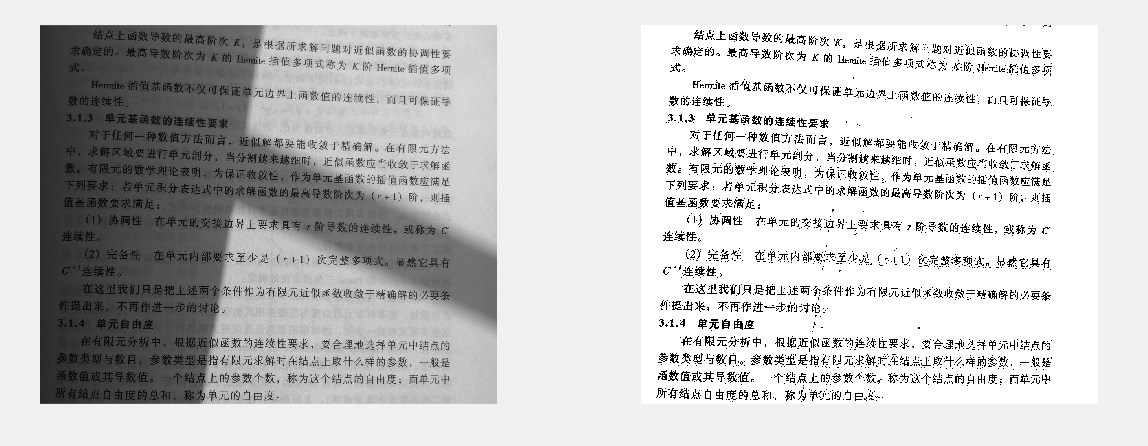## opencv实现二值图像孔洞填充

2018-04-22 11:45:37 jkjj2015 阅读数 9686
• ###### 书本封面文字识别

学完这门课程可以掌握C#数据库信息管理技巧和方法 掌握chart图表控件的使用 Excel数据的导出 两个日期间的数据查询，并生成图表

3人学习 金圣韬
免费试看

matlab中的imfill函数可以方便得实现二值图像的孔洞填充，而在opencv中并没有相同功能的函数。因此，在opencv的基础上编写实现孔洞填充的函数，并且能够设定阈值，对面积大于阈值的孔洞不进行填充。使用形态学重建的算法能够有效地实现孔洞填充，具体算法参照《数字图像处理》第三版9.5.9节，孔洞填充。

主要实现代码如下所示：其中imfill函数即为空洞填充的实现函数，第一个参数是二值图像（0~1），第二个参数是填充孔洞的阈值。若孔洞面积大于阈值则不填充，反之则填充。

#include "iostream"
#include <opencv2\opencv.hpp>
using namespace std;
using namespace cv;
Mat inv_board(Mat src);
Mat inv_img(Mat src);
void delarea(Mat& bw, int max);
Mat imfill(Mat I, int max);
void main()
{
Mat I, src_gray, F_B, F_BI_C, temp, H, I_fill;
cvtColor(scr, src_gray, COLOR_BGR2GRAY);
threshold(src_gray, I, 0.1, 1,0);
I_fill = imfill(I,40);
imshow("原二值图", I * 255);
imshow("填充图", I_fill*255);
waitKey(0);
}

Mat imfill(Mat I,int max)
{
Mat  src_gray, F_B, F_BI_C, temp, H, I_fill;
I_fill = I.clone();
Mat F = inv_board(I);
Mat I_C = inv_img(I);
Mat element = getStructuringElement(0, Size(3, 3), Point(1, 1));
while (1)
{

dilate(F, F_B, element);
F_BI_C = F_B.mul(I_C);
temp = F_BI_C - F;
if (sum(temp) == Scalar(0))
break;
else
F = F_BI_C.clone();
}
H = inv_img(F_BI_C);
Mat H_IC = H.mul(I_C);
delarea(H_IC, max);
for (int i = 0; i < H_IC.rows; i++)
{
for (int j = 0; j < H_IC.cols; j++)
{
if (H_IC.at<uchar>(i, j) == 1)
I_fill.at<uchar>(i, j) = 1;
}
}
return I_fill;
}
Mat inv_board(Mat src)
{

int rows = src.rows;
int cols = src.cols;
Mat dst = Mat::zeros(rows, cols, CV_8UC1);
for (int i = 0; i < cols; i++)
{
dst.at<uchar>(0, i) = 1 - src.at<uchar>(0, i);
}
for (int i = 0; i < cols; i++)
{
dst.at<uchar>(rows-1, i) = 1 - src.at<uchar>(rows - 1, i);
}
for (int i = 1; i < rows-1; i++)
{
dst.at<uchar>(i, 0) = 1 - src.at<uchar>(i, 0);
}
for (int i = 1; i < rows - 1; i++)
{
dst.at<uchar>(i, cols-1) = 1 - src.at<uchar>(i, cols-1);
}
return dst;
}

Mat inv_img(Mat src)
{
int rows = src.rows;
int cols = src.cols;
Mat dst = src.clone();
for (int i = 0; i < rows; i++)
for (int j = 0; j < cols; j++)
dst.at<uchar>(i, j) = 1 - src.at<uchar>(i, j);
return dst;
}

void delarea(Mat& bw, int max )
{
Mat bw_copy = bw.clone();
int flag = 0;
Mat H_b, H_bw, temp;
Mat H = Mat::zeros(bw.size(), bw.type());
for (int i = 0; i < bw.rows; i++)
{
for (int j = 0; j < bw.cols; j++)
{
if (bw_copy.at<uchar>(i, j) == 1)
{
H.at<uchar>(i, j) = 1;
Mat element = getStructuringElement(0, Size(3, 3), Point(1, 1));
while (1)
{
dilate(H, H_b, element);
H_bw = H_b.mul(bw);
temp = H_bw - H;
if (sum(temp) == Scalar(0))
break;
else
H = H_bw.clone();
}
bw_copy = bw_copy - H_bw;
if (sum(H_bw).val > max)
{
bw = bw - H_bw;
}
H = Mat::zeros(bw.size(), bw.type());
}

}
}
}